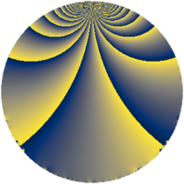# Properties

 Label 120.2.vLevel $120$ Weight $2$ Character orbit 120.v Rep. character $\chi_{120}(43,\cdot)$ Character field $\Q(\zeta_{4})$ Dimension $24$ Newform subspaces $1$ Sturm bound $48$ Trace bound $0$

# Learn more about

## Defining parameters

 Level: $$N$$ $$=$$ $$120 = 2^{3} \cdot 3 \cdot 5$$ Weight: $$k$$ $$=$$ $$2$$ Character orbit: $$[\chi]$$ $$=$$ 120.v (of order $$4$$ and degree $$2$$) Character conductor: $$\operatorname{cond}(\chi)$$ $$=$$ $$40$$ Character field: $$\Q(i)$$ Newform subspaces: $$1$$ Sturm bound: $$48$$ Trace bound: $$0$$

## Dimensions

The following table gives the dimensions of various subspaces of $$M_{2}(120, [\chi])$$.

Total New Old
Modular forms 56 24 32
Cusp forms 40 24 16
Eisenstein series 16 0 16

## Trace form

 $$24q + 4q^{6} - 12q^{8} + O(q^{10})$$ $$24q + 4q^{6} - 12q^{8} + 8q^{10} - 8q^{12} - 20q^{16} + 8q^{17} - 20q^{20} - 28q^{22} - 8q^{25} - 16q^{26} + 4q^{28} + 8q^{30} + 20q^{32} - 48q^{35} + 4q^{36} + 40q^{38} + 8q^{40} + 20q^{42} - 32q^{43} + 48q^{46} + 16q^{48} + 56q^{50} - 16q^{51} - 48q^{52} - 32q^{56} - 12q^{58} + 20q^{60} - 16q^{62} - 8q^{65} - 24q^{66} + 48q^{67} + 72q^{68} + 4q^{70} + 12q^{72} - 40q^{73} + 48q^{76} - 24q^{78} + 76q^{80} - 24q^{81} + 24q^{82} + 80q^{83} - 32q^{86} + 12q^{88} - 12q^{90} + 64q^{91} + 16q^{92} - 44q^{96} - 24q^{97} - 88q^{98} + O(q^{100})$$

## Decomposition of $$S_{2}^{\mathrm{new}}(120, [\chi])$$ into newform subspaces

Label Dim. $$A$$ Field CM Traces $q$-expansion
$$a_2$$ $$a_3$$ $$a_5$$ $$a_7$$
120.2.v.a $$24$$ $$0.958$$ None $$0$$ $$0$$ $$0$$ $$0$$

## Decomposition of $$S_{2}^{\mathrm{old}}(120, [\chi])$$ into lower level spaces

$$S_{2}^{\mathrm{old}}(120, [\chi]) \cong$$ $$S_{2}^{\mathrm{new}}(40, [\chi])$$$$^{\oplus 2}$$